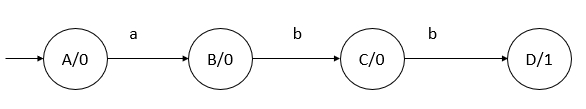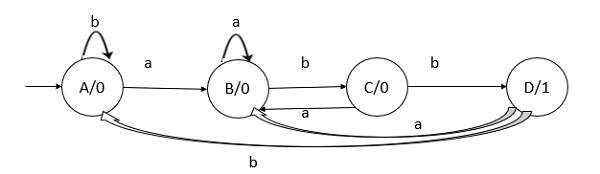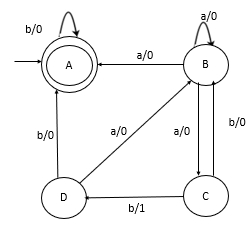# Convert the given Moore machine counts into equivalent Mealy machine.

Moore machine described by 6 tuples

(Q, q0, Σ, O, δ, λ)

Where,

• Q: Finite set of states
• q0: Initial state of machine
• Σ: Finite set of input symbols
• O: Output alphabet
• δ: Transition function where Q × Σ → Q
• λ: Output function where Q → O

Given Σ ={a,b} and Δ ={0,1}

Sequence= ‘abb’

Partial Moore machine with sequence ‘abb’ is as follows −The Full Moore Machine is as followsThe transition tables for Moore and Mealy Machines are as follows −

Transition table for Moor Machine −

Stateabo/p
ABA0
BBC0
CBD0
DBA1

Transition table for Mealy Machine −

Stateab
StateO/pStateO/p